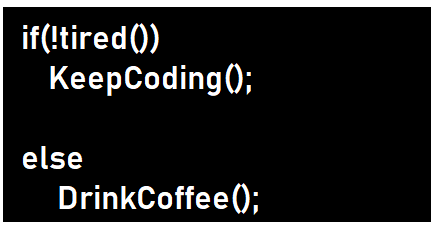# Types Of Recursion

## Introduction

Recursion is the process or a technique of defining a problem in terms of itself. It comes directly from Mathematics, where there are many examples of expressions written in terms of themselves.

In other words, we can say that it is a process in which a function calls itself directly or indirectly and that function is known as a recursive function.

It plays an important role in our day-to-day life. We can solve many exciting and important problems through recursion like Fibonacci NumberTower of Hanoi, DFS Traversal, and many more.

Recursion is needed for solving bigger problems that can be broken down into smaller, repetitive problems.

Recursion is divided into some types based on the calling nature of the function.

## Types of Recursion

There are two main types of recursion which depend on whether the function calls itself from within itself or whether two functions call one another mutually. The former is known as Direct recursion, and the latter is called indirect recursion.

Categories of recursion are:

1. Direct recursion
2. Indirect recursion

## Direct Recursion

Direct recursion can be further classified into four types:

Let's see all of them in detail for a better understanding.

## Tail Recursion

It is a special form of recursion where the function's last statement is the recursive call that calls itself. After that call, the function performs nothing. Tail recursion is better as the compiler can optimize it by executing the call in the current stack frame and returning its result rather than creating a new stack frame.

Let me explain to you a short example for a stronger building of approach.

For example, If we define the operation "find your way home" as:

1. If you are at home, stop moving.
2. Take one step toward home.

The solution is to reach the home in two steps.

1. We don't go home if we are already home.
2. We do an effortless action that makes our situation simpler to solve.
3. We redo the entire algorithm.

It is an example of tail recursion as the last statement is the recursive function calling itself.

Code

``````// Java code Showing Tail recursion
class Solution {

// Recursion function
static void func(int n){
// Base Case
if (n > 0){

System.out.print(n + " ");

// Last statement in the function
func(n - 1);
}
}

// Main function
public static void main(String[] args){
int n = 5;
func(n);
}
}``````

Output

Recursive tree representation for a better understanding of the above example.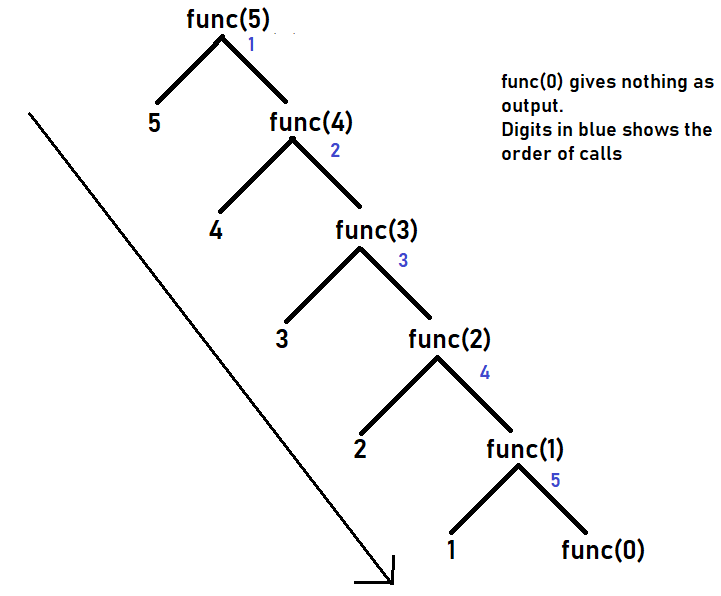Outputs are produced according to the order of the calls made.

Time Complexity: For tail recursion- O(n).

Space Complexity: For tail recursion- O(n).

Tail recursion can be converted into loops. Let's compare the codes and the space and time complexity to check which is more efficient.

Code

``````// Converting Tail Recursion into Loop
import java.io.*;
class Solution{

static void func(int n){
while (n > 0) {

System.out.print(" "+ n);
n--;
}
}

// Main function
public static void main(String[] args){
int x = 5;
func(x);
}
}``````

Output

Time Complexity: O(n)

Space Complexity: O(1)

It is better to write in a loop instead of tail recursion as space complexity is O(1) more efficient.

If the recursive function calls itself and that recursive call is the first statement, it is known as Head recursion. It is the opposite of tail recursion. There is no operation or statement before the function call, and all the processing has been done at the returning time, i.e., after the recursive call.

Code

``````import java.io.*;

class Solution {

// Recursive function
static void func(int n) {
if (n > 0) {

// First statement
func(n - 1);

System.out.print(" "+ n);
}
}

// Main function
public static void main(String[] args) {
int n = 5;
func(n);

}
}``````

Output

Time Complexity: For head recursion- O(n).

Space Complexity: For head recursion- O(n).

Recursive tree for the same example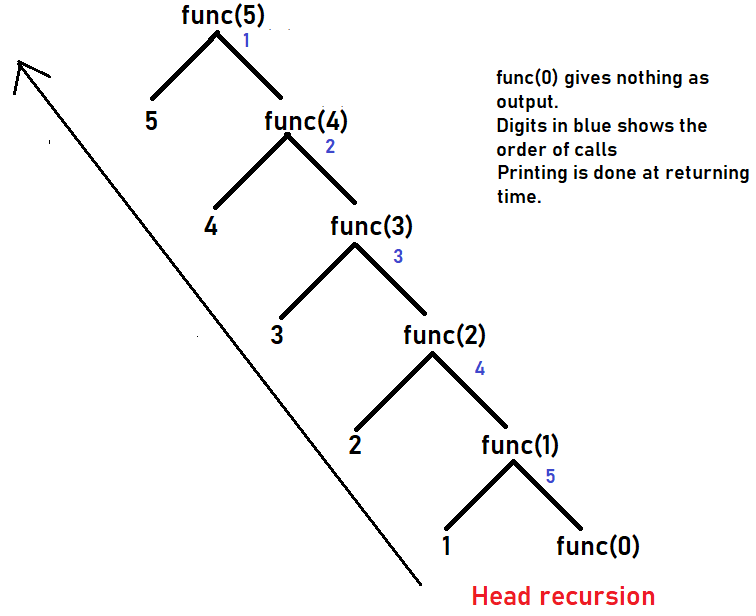Convert head recursion into a loop

Code

``````import java.util.*;
class Solution {
// Recursive function
static void func(int n) {
int i = 1;
while (i <= n) {
System.out.print(" "+ i);
i++;
}
}

// Main function
public static void main(String[] args) {
int n = 5;
func(n);
}
}``````

Output

• By looking at the recursive function, we can not convert it into a loop easily.
• We have to use another logic to perform the same operation.

## Tree Recursion

Before understanding tree recursion, do you have an idea about linear recursion?

If a recursive function calls itself only one time, then it is known as linear recursion.

If the recursive function calls itself more than once, it is known as tree recursion.

Code

``````class Solution {

// Recursive function
static void func(int n) {
if (n > 0) {
System.out.print(" "+ n);
func(n - 1);

// Calling the same function for the second time
func(n - 1);
}
}

// Main function
public static void main(String[] args) {
func(4);
}
}``````

Output

Tracing tree for better understanding of the example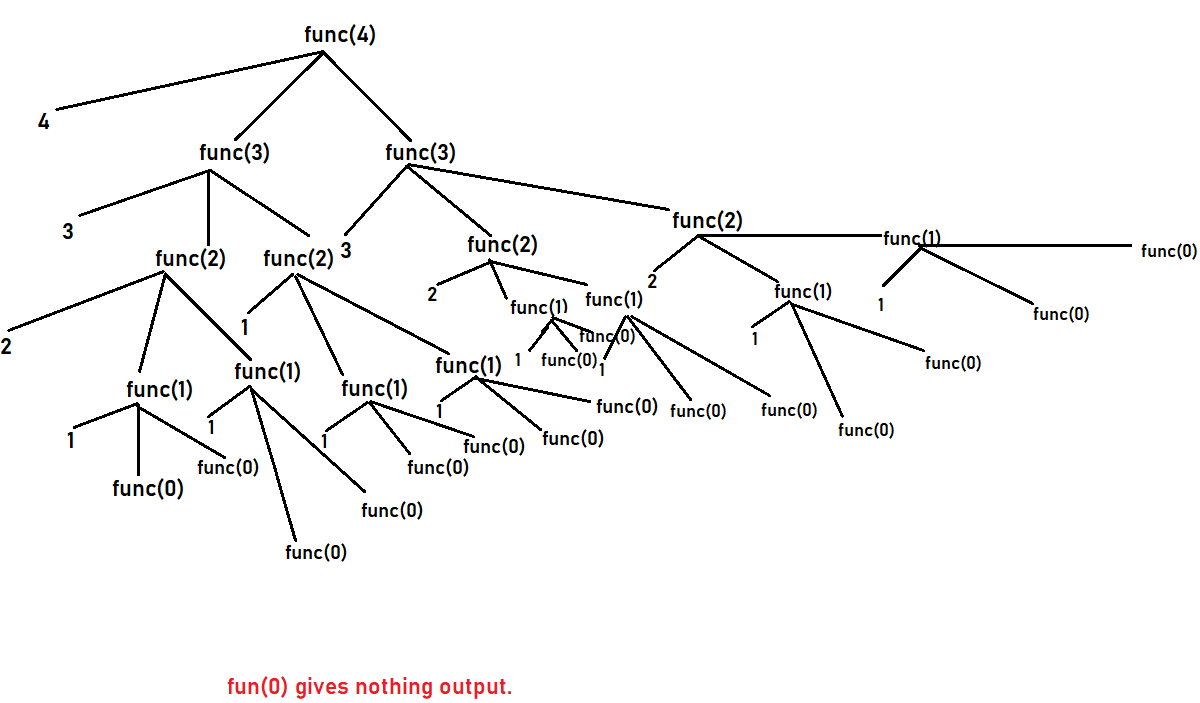Time Complexity: For tree recursion- O(2^n).

Space Complexity: For tree recursion- O(n).

## Nested Recursion

A recursive function where the argument passed to the function is the function itself.

Let's see an example to get more transparency regarding this recursion.

``````import java.util.*;

class Solution {
static int func(int n) {
if (n > 100){
return n - 10;
}

//recursion inside the recursion
return func(func(n + 11));
}

//Main function
public static void main(String args[]) {
int x;
x = func(95);
System.out.print(" "+ x);

}
}``````

Output

## Indirect Recursion

It is also known as mutual recursion. It occurs when the function calls another function, and that function eventually calls the original function.

The indirect recursion does not make any overhead as direct recursion.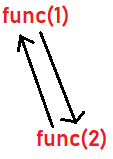Here, func(1) calls for func(2), and func(2) calls for func(1), which results in the formation of the cycle and eventually calling of the original function.

Code

``````// Java program to show Indirect Recursion
import java.io.*;

class Solution{

static void func1(int n) {
if (n > 0) {
System.out.print(" " +n);
// func(1) is calling func(2)
func2(n - 1);
}
}

static void func2(int n) {
if (n > 1) {
System.out.print(" " +n);

// func(2) is calling func(1)
func1(n / 2);
}
}

// Main function
public static void main (String[] args) {
func1(30);
}
}``````

Output

Tracing tree for better understanding1). What is the difference between recursive and iterative programming?

Recursive:

• Function calls itself.
• To find the time complexity is difficult.
• It has a large amount of overhead.
• Smaller code size.

Iterative:

• A set of instructions is executed.
• Lower time complexity.
• Less amount of overhead as compared to recursion.
• Larger code size.

2). How are stacks used in recursion?

Stack is used to store and restore the recursive function and its argument(s). To divide a problem into smaller pieces until reaching solvable parts. Then, solve the problem by solving these small pieces of the problem and merging the solutions.

3). What are the types of recursion in Data Structures?

There are mainly two types of recursion: Direct and Indirect.

## Key Takeaways

In this blog, we discuss various types of recursion with their examples in the Java language. For more information about recursion, you can check out here

You can also have a view of the Data Structures and Algorithms-guided path to start your preparation from scratch.